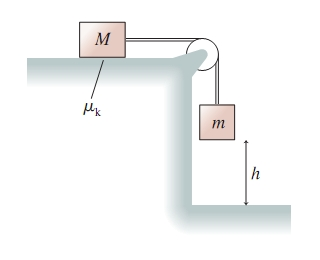# Problem: Use work and energy to find an expression for the speed of the block in the following figure just before it hits the floor.Find an expression for the speed of the block if the coefficient of kinetic friction for the block on the table is k.Find an expression for the speed of the block if the table is frictionless.

⚠️Our tutors found the solution shown to be helpful for the problem you're searching for. We don't have the exact solution yet.

###### Problem Details

Use work and energy to find an expression for the speed of the block in the following figure just before it hits the floor.Find an expression for the speed of the block if the coefficient of kinetic friction for the block on the table is k.

Find an expression for the speed of the block if the table is frictionless.